# Equations with Inequalities Solving Equations with Inequalities is

• Slides: 9Equations with Inequalities Solving Equations with Inequalities is just like solving any other equations that have variables. The main difference is that a regular equation with a variable has only one answer, an equation with an inequality has a range of answers. Regular Equation Inequality Equation x-4=3 +4+4 x-4>3 +4+4 x=7 x>7 The answer is one distinct number The answer is the range of numbers greater than seven Let’s graph this answer on a number line. 5 7 9 11 13 15 17 Notice the bold section of the line and that the circle on the seven is open.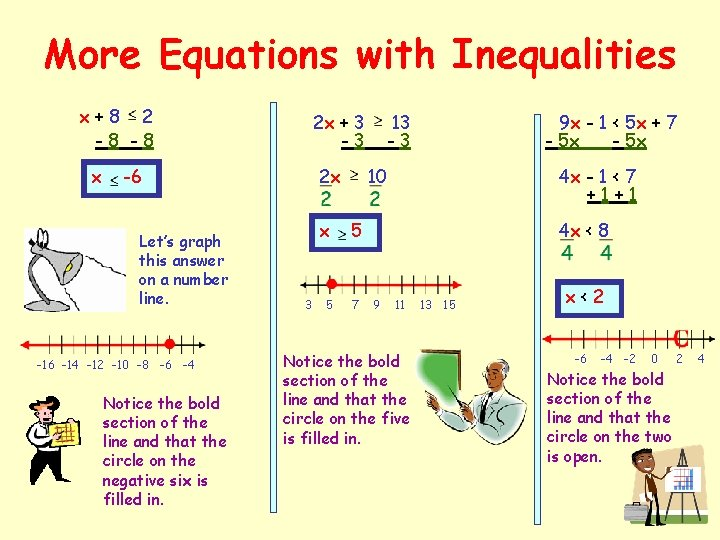More Equations with Inequalities x+8 2 -8 -8 x 2 x + 3 -3 -6 Let’s graph this answer on a number line. -16 -14 -12 -10 -8 -6 -4 Notice the bold section of the line and that the circle on the negative six is filled in. 2 x x 3 5 13 -3 9 x - 1 < 5 x + 7 - 5 x 10 4 x - 1 < 7 +1+1 5 7 4 x < 8 9 11 Notice the bold section of the line and that the circle on the five is filled in. 13 15 x<2 -6 -4 -2 0 Notice the bold section of the line and that the circle on the two is open. 2 4Absolute Value Inequalities That means the distance from zero is more than 2 units. That means the distance from zero is less than 2 units. -3 -2 -1 0 1 2 3 x is between the points. then If x is split on either side of the points. then If or Between SplitSolving Absolute Value Inequalities Less than means between Remove the absolute value symbol and write the equation according to the rules. Solve both sides of the inequality at the same time. Graph your solution on a number line. -4 -3 -2 -1 0 1 2 3 4 5 That was easy Write your answer in set form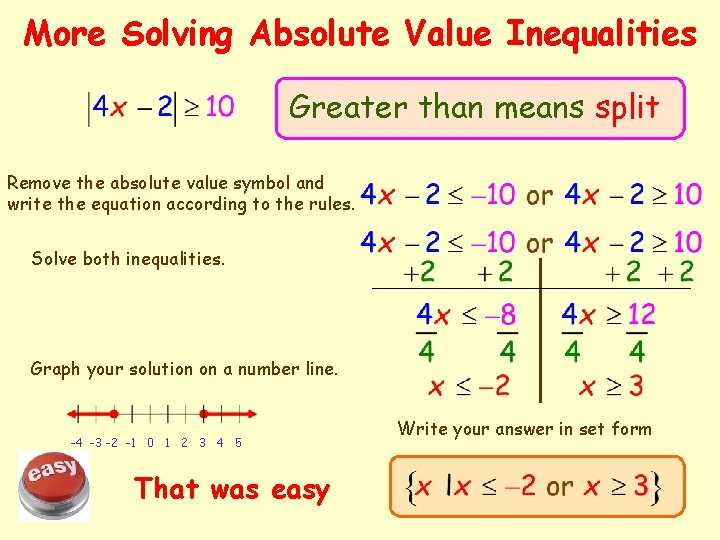More Solving Absolute Value Inequalities Greater than means split Remove the absolute value symbol and write the equation according to the rules. Solve both inequalities. Graph your solution on a number line. -4 -3 -2 -1 0 1 2 3 4 5 That was easy Write your answer in set form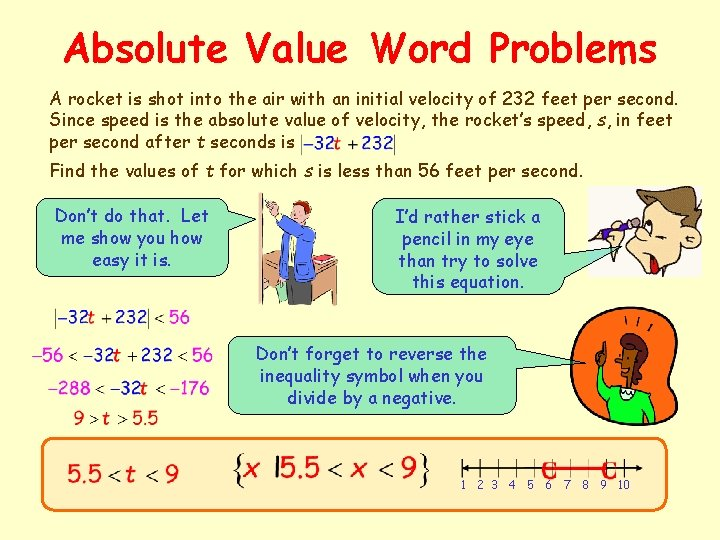Absolute Value Word Problems A rocket is shot into the air with an initial velocity of 232 feet per second. Since speed is the absolute value of velocity, the rocket’s speed, s, in feet per second after t seconds is Find the values of t for which s is less than 56 feet per second. Don’t do that. Let me show you how easy it is. I’d rather stick a pencil in my eye than try to solve this equation. Don’t forget to reverse the inequality symbol when you divide by a negative. 1 2 3 4 5 6 7 8 9 10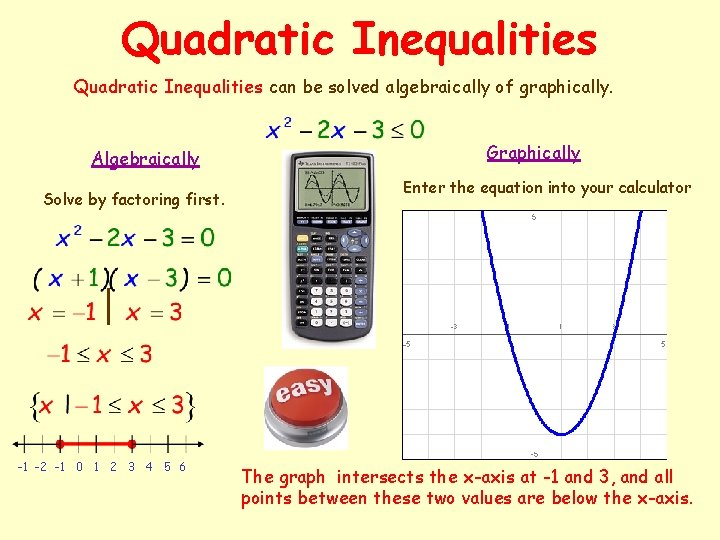Quadratic Inequalities can be solved algebraically of graphically. Algebraically Solve by factoring first. -1 -2 -1 0 1 2 3 4 5 6 Graphically Enter the equation into your calculator The graph intersects the x-axis at -1 and 3, and all points between these two values are below the x-axis.More Quadratic Inequalities Algebraically Graphically Reverse the inequality symbol when you divide by a negative. Greater than means split. -5 -4 -3 -2 -1 0 1 2 3 The graph intersects the x-axis at -4 and 2, and all points between these two values are above the x-axis.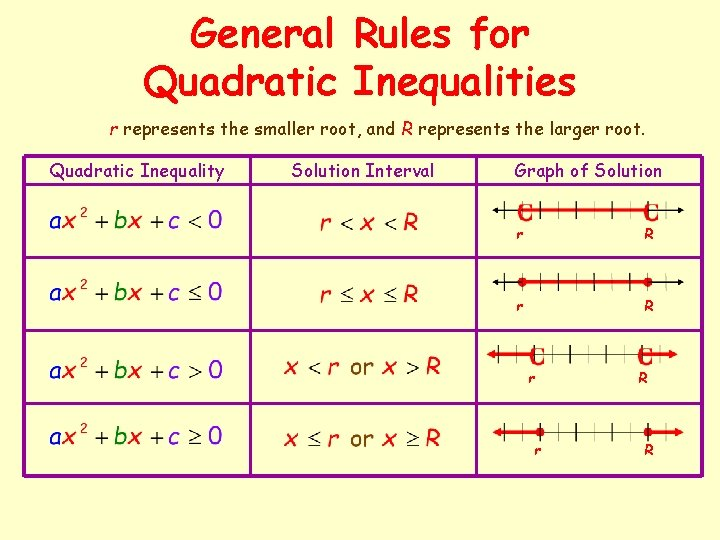General Rules for Quadratic Inequalities r represents the smaller root, and R represents the larger root. Quadratic Inequality Solution Interval Graph of Solution r R r r R R